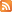Entries (RSS) -Comments (RSS)

### Elegant Algebraic Equations for 3D prisms

Shape                                      Formula
Cube                                        A = 6s2                            Where s is a side length.

Rectangular Prism                  A = 2B + Ph
Where B is the area of the base, P is the perimeter of the base and
h is the height.

A = 2(lw + lh + wh)
Where l is the length, w is the width,
and h is the height.

Pyramid                                  A = 4[(bh)/2] + lw
Where b is the base length, h is the height of the triangle (the slant
height), l is the length of the base, and w is the width of the base.

A = B + ½(Ph)
Where B is the area of the base, P is the perimeter of the base,
and h is the height of the triangle (the slant height).

Isosceles triangular prism             A = 2[(b1h1)/2]  + 2[(b2h2)/2] + B
Where b1 and h1 is the base and height related to the triangle,
b2 and h2 is the base and height related to the rectangles,

and B is the area of the base.

Cylinder                                          A = 2πr2 + (2πr)h
A = 2πr(r + h)

Where r is the radius of the circle.

Where B is found by finding the area of the base (length x width),
and P is the sum of all sides of the base.

#### Comments

No responses to “Elegant Algebraic Equations for 3D prisms”